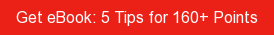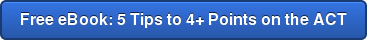# SAT / ACT Prep Online Guides and TipsHow exactly do you take a document that's as complicated as your transcript and shrink it all down to a single number? If you're wondering how to use the final grades you've gotten in high school to determine your GPA, then you've come to the right place. This article will show you how to make this calculation, step by step. But first, what exactly is a GPA?

## What Is a GPA?

Most likely, in your high school classes, your final grades are awarded either as letters (A-, B+, etc.) or percents (92%, 85%, etc. out of 100%).

A GPA, or grade point average, converts those letters or percents into numbers and then averages these numbers together. Because it's made up of all your grades, your GPA is one of the most important factors for college admission. It's a good indicator of your intelligence, work ethic, perseverance, and willingness to push yourself.

GPAs are useful for colleges to easily compare you with other students who graduated from your school and with all the other applicants. But why?

Imagine you're an admissions officer who has to look at thousands of college applications. Would you rather go through each transcript individually, add up all the As and all the Bs, and then compare that to the next person, and so on? Or, would you rather have an easy summary number that could be used for a quick comparison across the board?

Your GPA is that quick summary number.Turn the hard to eat ingredients of your transcript into the gooey s'more deliciousness of a GPA. Probably not with an open flame, though.

## The Difference Between Weighted and Unweighted GPA

There are two main types of GPAs: weighted and unweighted.

An unweighted GPA is when a school uses a scale that goes from 0.0 to 4.0 and does not take into account the difficulty level of classes.

By contrast, a weighted GPA is when a school uses a scale that goes from 0.0 all the way up to 5.0 (or sometimes 6.0) and does take into account class difficulty. In this model, the school gives higher numerical values to grades earned in honors, AP, and/or IB classes.

Here's an example to help clarify the differences here. Say Jeremy gets an A in a standard-level US History class, whereas Lakshmi gets an A in AP US History. In the unweighted GPA model, both As are treated the same way, with each translating to a 4.0.

But in the weighted GPA model, Jeremy's A would convert to a 4.0 and Lakshmi's A would convert to a 5.0 to show that her class took a lot more effort to ace.

See the difference?

Before we continue, it's important to understand that this article focuses mainly on explaining and calculating unweighted GPAs. (For more information on weighted GPAs, check out our other guide.)Sure, you can pick them up, but doesn't it help to know how much each weighs?

## How Do You Calculate Your Unweighted GPA?

The first thing to do in order to calculate a grade point average is to convert each of the final class grades you've gotten so far in high school into the correct decimal.

Here is the standard unweighted scale for doing this:

 Letter Grade Percentage GPA A+ 97-100 4.0 A 93-96 4.0 A- 90-92 3.7 B+ 87-89 3.3 B 83-86 3.0 B- 80-82 2.7 C+ 77-79 2.3 C 73-76 2.0 C- 70-72 1.7 D+ 67-69 1.3 D 65-66 1.0 F Below 65 0.0

Next, perform the following calculation:

• Count the number of classes you've taken
• Divide the sum by the number of classes, and you have your unweighted GPA

In the following section, we'll go through an example calculation of an unweighted GPA.Want to build the best possible college application?

We can help. PrepScholar Admissions is the world's best admissions consulting service. We combine world-class admissions counselors with our data-driven, proprietary admissions strategies. We've overseen thousands of students get into their top choice schools, from state colleges to the Ivy League.

We know what kinds of students colleges want to admit. We want to get you admitted to your dream schools.## Step-by-Step Example of an Unweighted GPA Calculation

Let me show you an example of how to calculate an unweighted GPA so you can see how this will look in practice. (To see how to calculate a weighted GPA, check out our other article.) We'll use a sample transcript for incognito CIA operative John Doe.

Be aware that for this example, we are assuming that all classes are worth the same number of credits (in other words, you can ignore the credit column in the transcript below).### Step 1: Convert Grades Into Decimals

In order to learn how to calculate a GPA, let's first convert John's letter grades into numbers:

 9th Grade 10th Grade 11th Grade 12th Grade 3.0 4.0 4.0 3.0 4.0 3.0 2.7 3.0 3.3 3.7 3.7 4.0 3.7 4.0 3.0 3.0 3.0 4.0 3.3 3.0 3.3 3.3 4.0 3.7 4.0 4.0 4.0 3.7 4.0 3.0 3.0 2.7 4.0 3.0

Let's also count how many classes he took each of those school years:

### Step 2: Calculate Individual Year GPAs

To get each individual year's GPA, all we need to do is divide the sum by the number of classes. If this division ends up with a long decimal, simply round to the nearest hundredth:

 9th Grade 10th Grade 11th Grade 12th Grade 35 / 10 = 3.50 35.7 / 10 = 3.57 27.7 / 8 = 3.46 19.7 / 6 = 3.28

### Step 3: Calculate Cumulative High School GPA

To get a cumulative GPA for John's entire high school career, we simply add up the sums for all the years and divide by the number of classes he took over all those years:

35 + 35.7 + 27.7 + 19.7 = 118.1 (sum of all final grades)

10 + 10 + 8 + 6 = 34 (total number of classes taken)

118.1 / 34 = 3.47 (GPA)

So, his GPA for all of high school is 3.47.

Pro tip: The cumulative GPA is not an average of each year because the number of classes taken each year is different.

### Step 4: Calculate GPA Submitted to Colleges (Optional)

Finally, if we wanted to figure out the GPA that John would send out on his college applications, we would do the same process, but leave off senior year. Since applications go out in the beginning of 12th grade, those final grades won't make it into the application GPA:

35 + 35.7 + 27.7 = 98.4 (sum of final grades from 9th to 11th grade)

10 + 10 + 8 = 28 (number of classes taken from 9th to 11th grade)

98.4 / 28 = 3.51 (college application GPA)

As you can see, John's college application GPA would be 3.51.Is nicely done, John!

## What If My Classes Are Worth Different Amounts of Credits?

In the example above, we calculated John's GPA with the assumption that every took was worth the same amount of credits. If this isn't the case for you, you'll need to add in an extra step.

To calculate your GPA when your classes are worth different amounts of credits, you'll need to multiply your grade for each class by the number of credits it was worth and sum those together (instead of just summing all your grades together) and divide that sum by the total number of credits you took (rather than just the total number of classes you took).

As a quick example, let's take another look at John's junior year grades, this time with the number of credits each class was worth.

 GPA Credits Quality Points 4.0 1 4.0 2.7 1 2.7 3.7 1 3.7 3.0 1 3.0 3.3 1 3.3 4.0 1 4.0 4.0 0.5 2.0 3.0 0.5 1.5

Here we can see that the last two classes John took were each only worth half a credit. In the table, the third column, "Quality Points," shows the product of John's GPA in each class with the number of credits that class was worth.

To find John's GPA (based solely on these eight classes), all you need to do now is sum the number of quality points and divide them by the number of credits John took:

GPA = (4.0+2.7+3.7+3.0+3.3+4.0+2.0+1.5) / (1 + 1 + 1 + 1 + 1 + 1 + 0.5 + 0.5)

GPA = 24.2/7 = 3.46.

## What's Next?

Interested in diving even deeper into the differences between weighted and unweighted GPA? Then check out our guide to the benefits and drawbacks of both.

Want to see step-by-step weighted GPA calculation? Let us show you how it's done.

Curious how your GPA compares? Learn what a good or bad GPA is and then see how you stack up against the average high school student.

Want to improve your SAT score by 160 points or your ACT score by 4 points? We've written a guide for each test about the top 5 strategies you must be using to have a shot at improving your score. Download it for free now:Dr. Anna Wulick

Anna scored in the 99th percentile on her SATs in high school, and went on to major in English at Princeton and to get her doctorate in English Literature at Columbia. She is passionate about improving student access to higher education.

Student and Parent Forum

Our new student and parent forum, at ExpertHub.PrepScholar.com, allow you to interact with your peers and the PrepScholar staff. See how other students and parents are navigating high school, college, and the college admissions process. Ask questions; get answers.